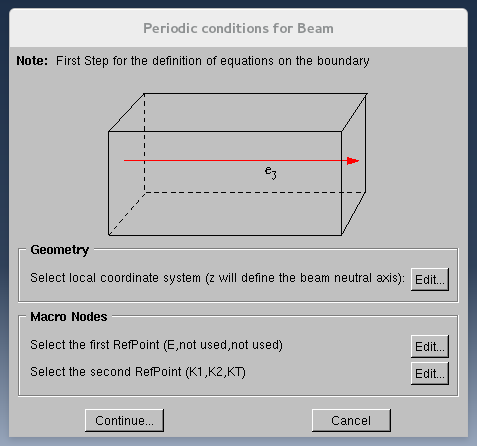]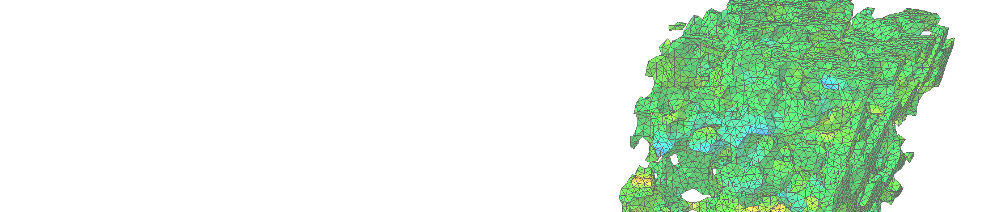### Heterogeneous Structures

Homtools can be used to estimate the average mechanical response of heterogeneous periodic beams or plates by the way of Finite Element Analysis on the 3D periodicity cell. It allows to compute the relationship between the average generalized stresses (normal stress, bending moments, twisting moment...) and the average generalized strains (normal strain, bending curvatures...). It can be used for example to estimate the effective inertia of the equivalent homogeneous beam in the linear elasticity framework. Nevertheless, the constitutive materials of the periodicity cell can be non-linear and the presence of interaction properties is naturally taken into account. Homtools can thus help to build a non-linear effective beam or plate model and identify its parameters.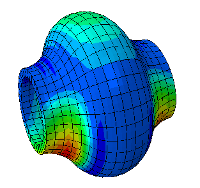# Heterogeneous Periodic Beams

This functionnality is relevant for heterogeneous beams that are periodic in the direction of their axis. In that case, a periodicity cell can be defined, together with a periodicity vector which is parallel to the beam axis. The kinematics of the effective beam model is the one of Navier-Euler-Bernoulli (no transverse shearing). Four effective generalized strains are considered : the normal strain, the two bending strains and the twisting strain. The associated effective generalized stresses are the normal stress, the bending moments and the twisting moment. Homtools allows to prescribe an average loading in terms of these effective strains or stresses (or any independent combination of them) on the 3D periodicity cell. It requires non-classical boundary conditions that can be written as linear constraints between the displacements at each point on the boundary of the periodicity cell and the average strains.

This method consists in applying periodicity conditions on the two opposite cross-sections of the periodicity cell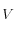. A local orthonormal coordinates system must be defined, such that the axis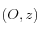is the beam reference axis. The normal strain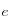characterize the effective elongation along. The bending strains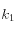and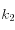are the effective curvature around the axis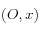and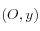and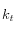is the twisting strain around. The effective stresses (normal stress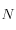, bending moment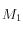and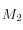, and twisting moment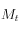) are defined as: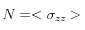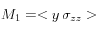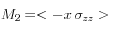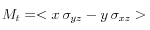in which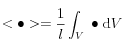with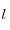the length of the periodicity cell (norm of the periodicity vector).

The periodic homogenisation process consists in assuming that the strain field is the sum of a pure Euler-Bernoulli-Navier strain field and of a periodic fluctuation strain field that takes into account the heterogenities. It leads to the following boundary conditions: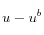periodic and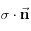antiperiodic,

in which: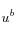is the displacement that leads to a pure Euler-Bernoulli-Navier strain field (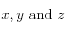are the coordinates of a current point) :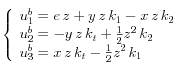a quantity is said to be "periodic" (resp. "antiperiodic") when it takes the same value (resp. opposite value) at two opposite points on the boundary (one of them being the image of the other by translation of a periodicity vector).

Homtools uses Reference Points to prescribe the average loading and these constraints.

# Heterogeneous Periodic Plates

Under construction

# Macro nodes and Reference axis or plane

As in the case of material homogeneisation, reference nodes needs to be added to the model. The degree of freedom of these nodes are associated to the generalized strains. Linear relation (*equation) will automatically be generated by Homtools between the reference nodes and all the mesh nodes that are selected by the users as boundary nodes. However, users also need to define an axis (for beam) or a plane (for plates) which will be used as the neutral axis or plane to define the effective generalzed strains and stresses.

Here is a sample of the GUI for beam: